# Anamorphic 3D Printing

You’ve seen funny mirrors at carnivals, the curvy ones that make your head look tiny and your legs impossibly long. Now imagine a person who looks strange in real life, say with a giant head and extremely short legs. Put this unusual character in front of the same curvy mirror and now you see someone who looks normal. That’s the idea behind an anamorphic representation. In his follow-up to the last post, Paul Kassebaum shows us how to compute the inverse funhouse mirror effect for our old friend, the L-shaped membrane.

## Reflections in a Cylinder

by Paul Kassebaum

Using MATLAB to make models for 3D printing gives you the ability to perform all sorts of mathematical transformations to define or deform your creation.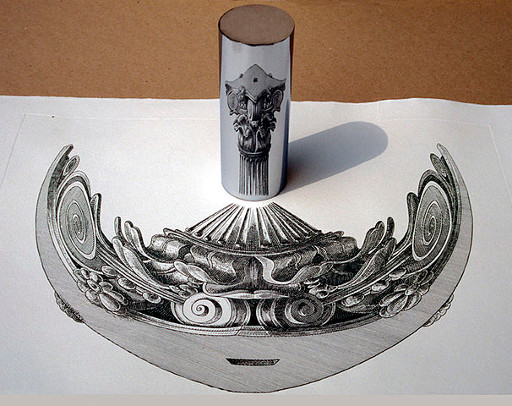Anamorphic drawing by Istvan Orosz, entitled Anamorph with column 2.

In my previous post, we explored how to turn equations and data into 3D prints, using the L-shaped membrane as an example. In this post, we’ll have a little physics fun with our model in MATLAB before sending it off to be printed, deforming it to create an anamorphic sculpture. In particular, we’ll create a 3D print of the L-shaped membrane that is meant to be viewed in the reflection of a cylinder.

### Contents

#### Underlying physics

To make an anamorphic sculpture look intelligible when reflected off a curved mirror, the only physics you need is the law of reflection. The law of reflection notes that if a ray of light hits a mirror at an angle measured off a line perpendicular to the mirror, then the reflected ray of light will come off the mirror at that same angle.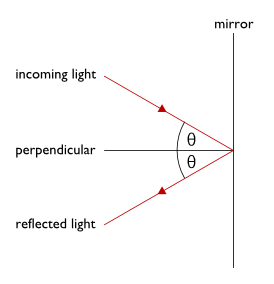Simple case of the law of reflection where the mirror is flat.

Reflections off flat mirrors create every-day optical illusions. The mirror appears to be a window to an ethereal world on its other side. However, every ray of light that appears to be coming from the other side of the mirror is in fact coming from the same side we are standing on, only bent at angles that we can calculate using the law of reflection.

To make our anamorphic sculpture work with a cylindrical mirror, we have to imagine that the L-shaped membrane is sitting on the other side of the window the mirror appears to make. This is the same as imagining that the sculpture is sitting in the center of the cylinder. Let me explain further by referencing the drawing below. For every point P on this apparent sculpture inside the cylinder, we’ll draw a straight line to the eye of the viewer at a point V. This line from P to V is the path that our eyes think a ray of light took. However, the light actually came from some point P’ on our side of the mirror, hit the mirror at some angle, reflected off the mirror at that same angle, and then hit our eyes.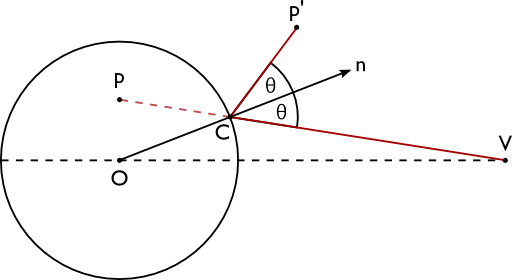Drawing of how light reflects off a cylinder from P’ to the viewer’s eye at V, creating the apparent image at P. The cylinder is shown from above.

Our task is to figure out where P’ is located given the location of P, V, and the radius of the cylinder. Since our sculpture of the L-shaped membrane is made up of triangular faces, we need to solve this problem for every triangle vertex.

Once the intersection points are found, the problem is essentially two dimensional. Each reflected pair of points P and P’ will have the same altitude. The rest of the problem focuses on lines and points that are projected onto a plane to create drawings like the one shown above.

#### The inverse reflection problem

This problem can be solved in just a few steps.

1. First, we’ll find the point of intersection C between the line PV and the cylinder.
2. Then we’ll find the angle between the line perpendicular to the cylinder and the line PV. This is the angle of the reflected ray of light shown in the drawing above.
3. Finally, we know that the light ray CP should have the same length as CP’, so we can find P’ by rotating the point P about C by an angle that satisfies the law of reflection.

#### The intersection points

Imagine a photon traveling along the line PV from P to V. This photon’s journey can be tracked by a vector J defined by position vectors P and V, and a factor t:

J = P + t*(V-P) where t is in the range [0,1],

so as t increases from 0 to 1, the photon moves from P to V.

The photon will hit the cylinder’s surface at some particular t. At this point, the position vector of the photon measured from the cylinder’s center will have the same length as the radius of the cylinder, or

$|J|^2 = R^2$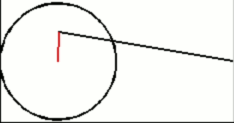Demonstration of the equality above.

This equality sets up a quadratic equation for the value of t at which J intersects the cylinder. This equation can be solved for t using the quadratic formula

A = p(:,1).^2 + p(:,2).^2 - 2*p(:,1)*v(1)...
+ v(1)^2    + v(2)^2    - 2*p(:,2)*v(2);
B = 2*(p(:,1)*v(1) + p(:,2)*v(2) - p(:,1).^2 - p(:,2).^2);
C = p(:,1).^2 + p(:,2).^2 - R^2;
t = (-B + sqrt(B.^2 - 4*A.*C))./(2*A);


The code above solves the problem for every point on the apparent model. The variable p is an n-by-3 matrix representing n points of the apparent model’s triangular vertices, each with 3 cartesian coordinates, and v is a 3 component vector representing the viewing point. In this way, t is now an n component vector with each component of t associated with each point in the matrix p. Notice that although the quadratic formula gives us two roots, we’ve chosen the one that guarantees that t is always positive.

The intersection points c are then

pv = bsxfun(@minus,v,p); % p to v
c  = p + bsxfun(@times,t,pv);


#### The angles of reflection

Now that we know the point of intersection C for each point on the apparent model P, we can find the angles of reflection. Since we would like to know both the angle and the orientation (clockwise or counterclockwise) to rotate the point P about C, we should use the ATAN2 function to calculate the angle between the position vector for C and the vector PC from P to C.

pc = c - p;
theta = atan2(c(:,2),c(:,1)) - atan2(pc(:,2),pc(:,1));


#### Locate the real model’s points

With the reflection angles in hand, we can now specify exactly where the points P’ must be. We’ll rotate the vector PC about C by twice the reflection angle: once to bring it along the vector to C, and once more in accordance with the law of reflection.

For each apparent point P, we will have a matrix equation

$p’ = R(\theta) * (c – p) + c,$

where R is a rotation matrix.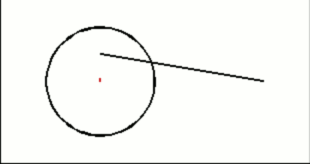To locate P’, draw position vectors to P and C, create the vector PC, rotate this vector by twice the angle calculated above, then translate the vector along C.

In code, we’ll first set up all of the rotation matrices

theta = 2*theta;
Rotate = [cos(theta) -sin(theta) zeros(numel(theta),1),...
sin(theta)  cos(theta) zeros(numel(theta),3),...
-ones(numel(theta),1)];% negative so
% that pp_z = p_z
Rotate = reshape(Rotate',3,3,[]); % 3-by-3-by-n


Then we’ll reshape our vectors and perform the rotations and translations.

p = reshape(p',3,1,[]); % 3-by-1-by-n
c = reshape(c',3,1,[]); % 3-by-1-by-n
pp = zeros(size(p)); % preallocate p'
for k = 1:size(p,3)
pp(:,:,k) = Rotate(:,:,k)*(c(:,:,k) - p(:,:,k)) + c(:,:,k);
end
% Reshape pp to bring it into the original form of p
pp = reshape(pp,3,[])'; % n-by-3


#### The big picture

Putting all of those steps together, we can define the following function

function pp = reflectThroughCylinder(R, v, p)
...% download this function from the File Exchange
end


Let’s put this function to use on the L-shaped membrane sculpture! Using the code explained in the previous post, we have the L-shaped membrane sculpture defined by the vertices of triangles stored in the variable shellVertices, and the way these vertices are connected stored in the variable shellFaces.

R = 2*sqrt(2)/2; % Twice the minimum radius to fit the apparent sculpture.
v = 1.5*R*[cos(0.9406),sin(0.9406),1]; % View from 1.5 radii away
% creates a nice distortion.
p = bsxfun(@minus,shellVertices,[0.5,0.5,0]);% Place origin at x-y
% center of sculpture.
pp = reflectThroughCylinder(R,v,p);

% Visualize the anamorphic sculpture
trisurf(shellFaces,...
pp(:,1),...
pp(:,2),...
pp(:,3));
colormap pink;
axis equal;
axis vis3d;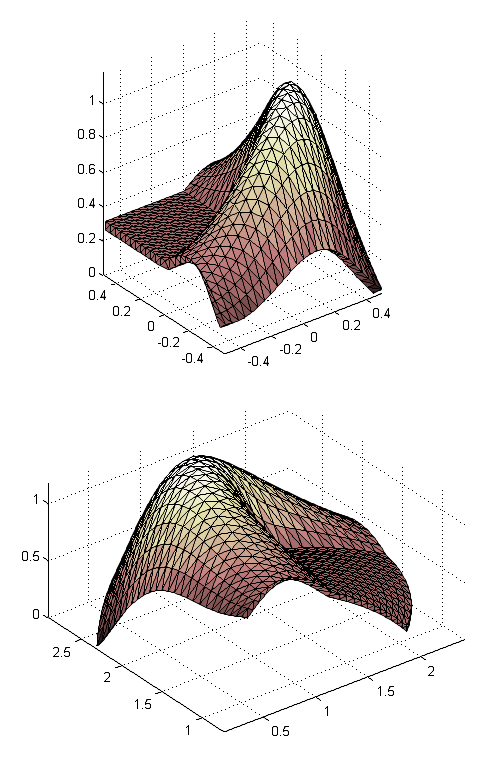The apparent and reflected L-shaped membranes.

Here’s the final product!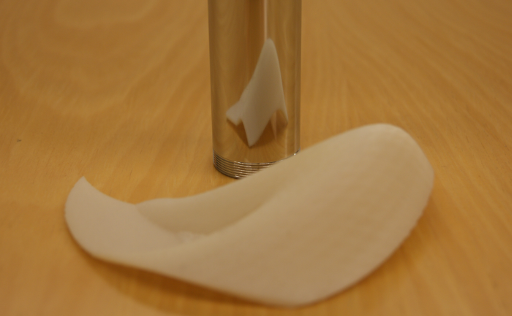Have fun making your own anamorphic sculptures to amaze your friends!

|